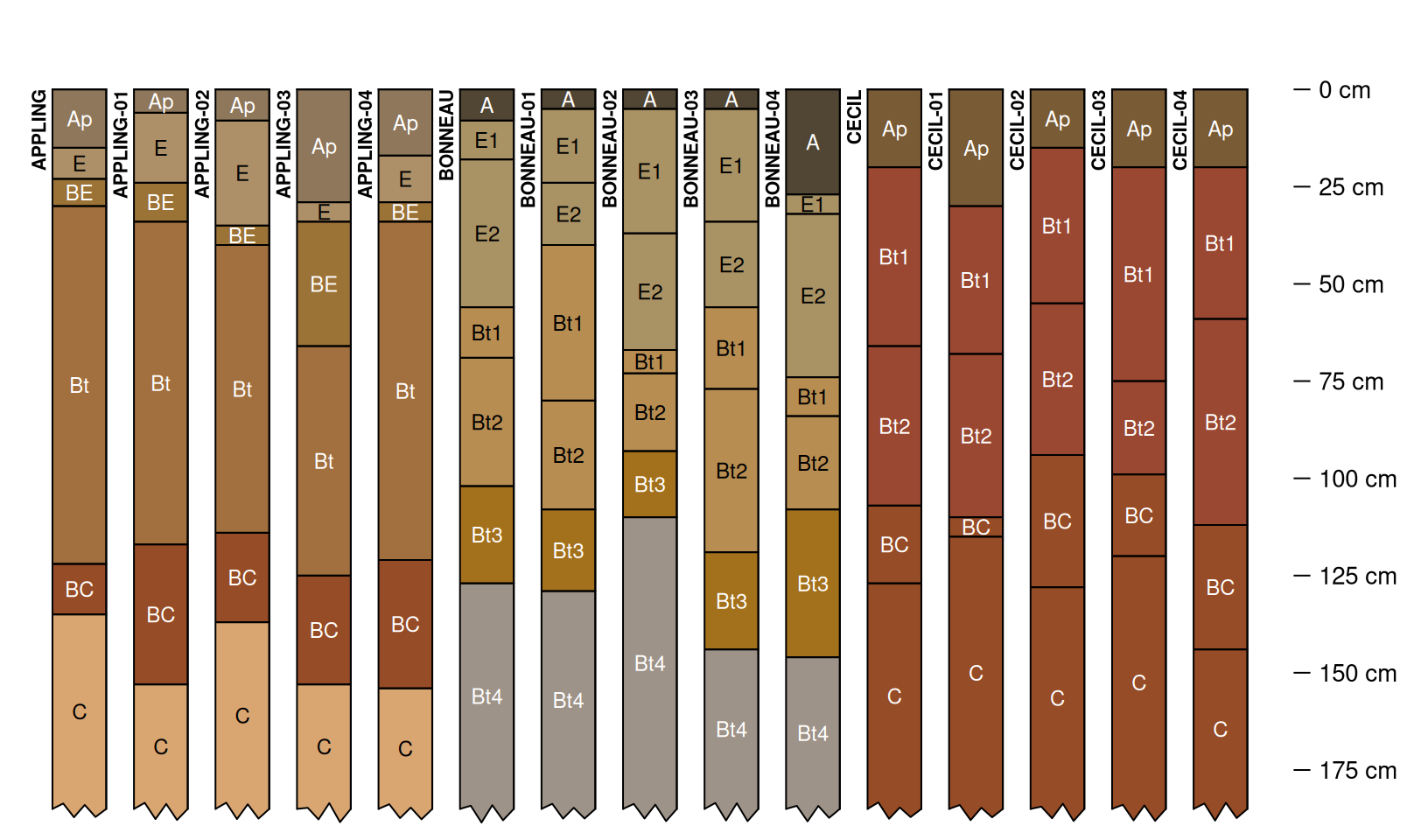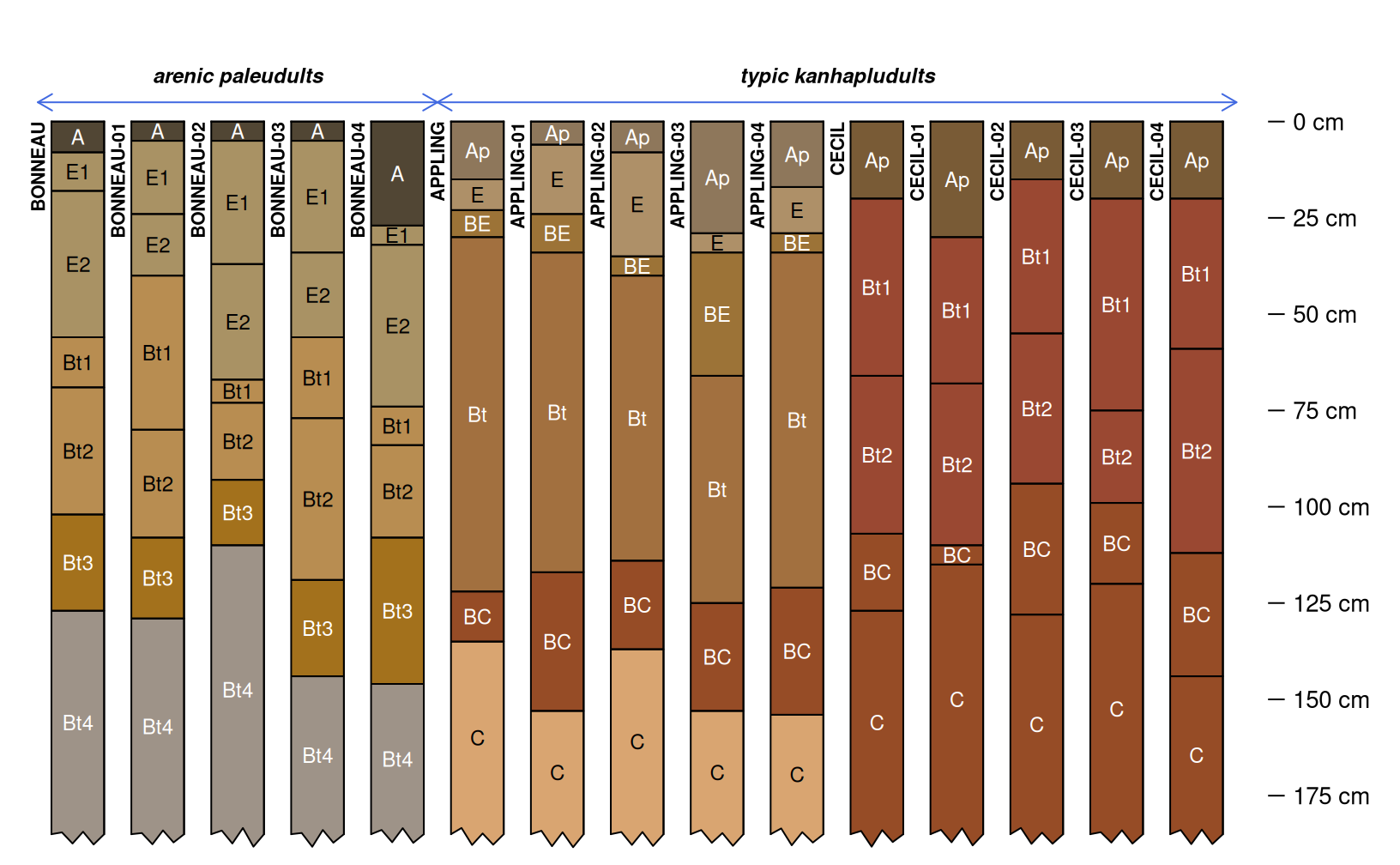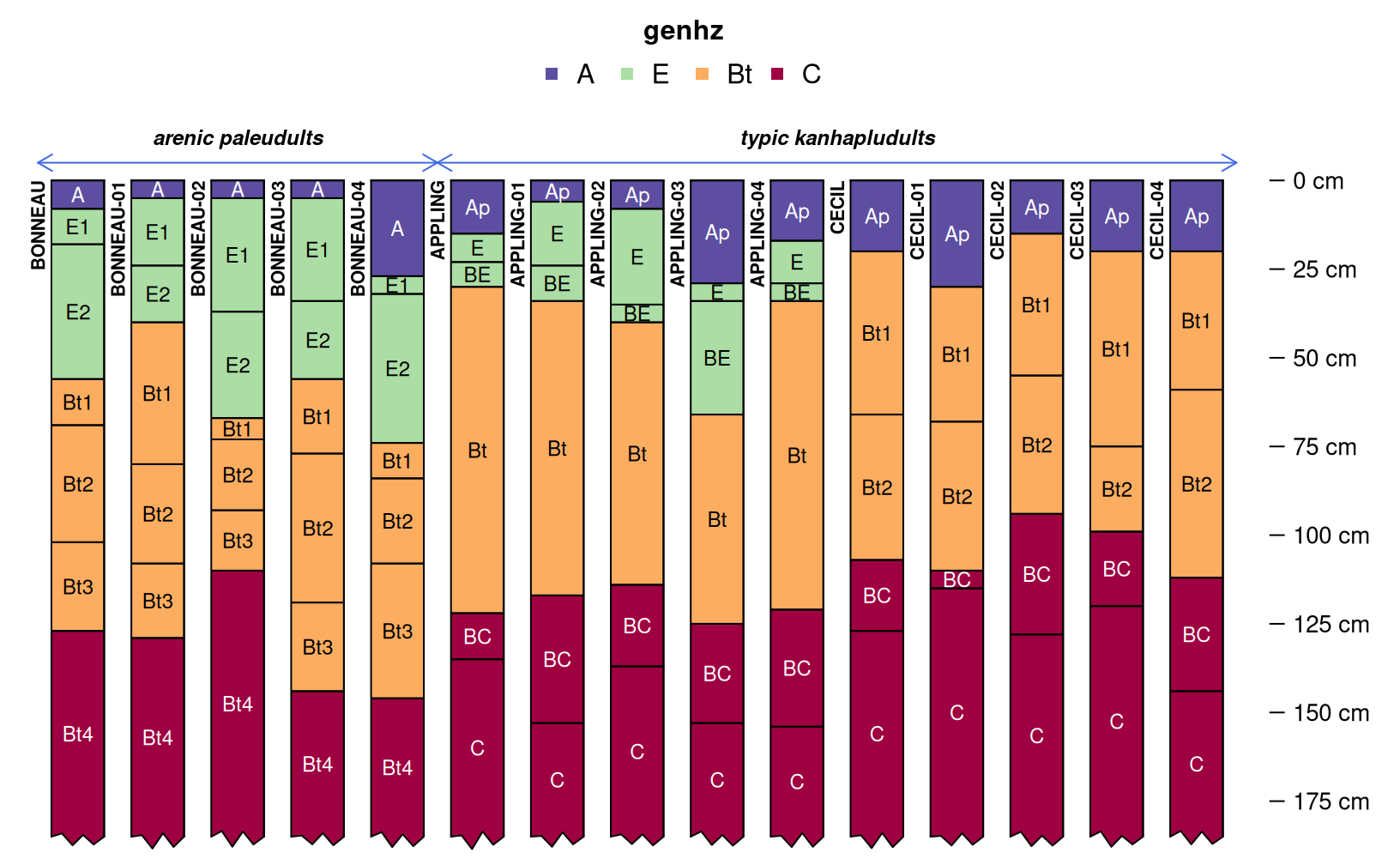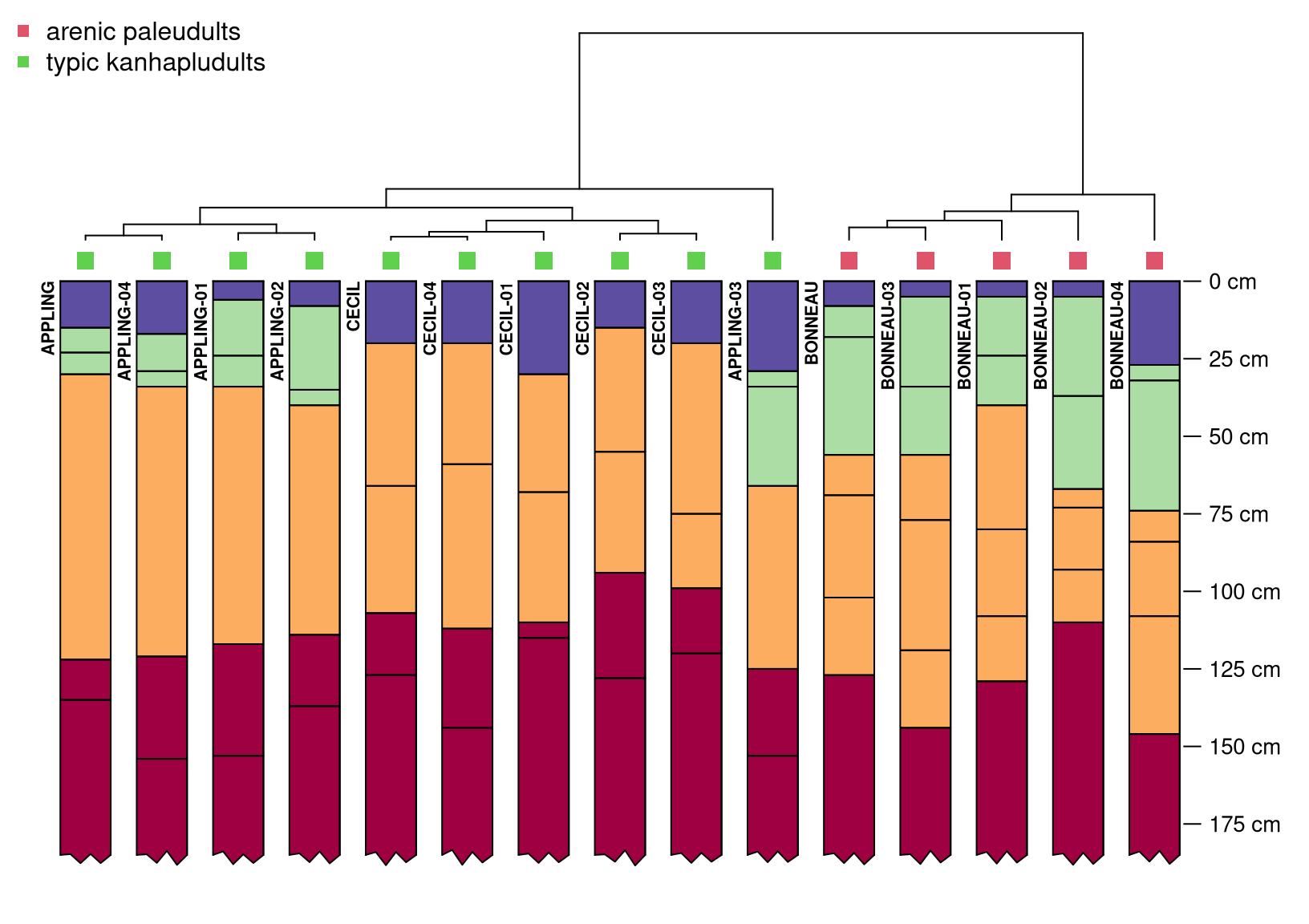## Introduction

With the release of {aqp} 2.0, the soil profile comparison algorithm implemented in profile_compare() (Beaudette et al., 2013) has been completely re-written as NCSP() and re-named the “Numerical Comparison of Soil Profiles”. A more recent discussion of this algorithm is provided in Maynard et al. (2020).

This short vignette demonstrates how to use the NCSP() function from {aqp} 2.x to perform a pair-wise comparison of soil data encoded as a SoilProfileCollection object. The pair-wise comparison of site-level attributes, previously available in profile_compare() has been removed from NCSP() and implemented as a stand-alone function named compareSites(). A final distance matrix (combining horizon and site level attributes) is created via weighted average. A more detailed version of this vignette can be found in the Pair-Wise Distances by Generalized Horizon Labels tutorial.

## A Simple Example

Consider three soil profiles, containing basic morphology associated with the Appling, Bonneau, and Cecil soil series. These data are provided in the example dataset “osd” as part of the {aqp} package.

library(aqp)
library(cluster)
library(sharpshootR)
library(ape)

# load data and make a copy
data("osd")
x <- osd

Simulation is used below to generate 4 realizations of each soil series, using the perturb() function.

# assume a standard deviation of 10cm for horizon boundary depths
# far too large for most horizons, but helps to make a point
x$hzd <- 10 # generate 4 realizations of each soil profile in x # limit the minimum horizon thickness to 5cm set.seed(10101) s <- perturb(x, id = sprintf("sim-%02d", 1:4), boundary.attr = 'hzd', min.thickness = 5) # combine source + simulated data into a single SoilProfileCollection z <- combine(x, s) A quick review of the source and simulated profiles, note patterns in horizon depths, horizon designation, and moist soil colors. The profiles have been visually truncated at 185cm for clarity (note ragged bottoms). The new .aqp.plotSPC.args option is used to set default arguments to plotSPC() for the remainder of the R session. Simulated profiles are labeled with a numeric suffix (e.g. “-01”) # set plotSPC argument defaults options(.aqp.plotSPC.args = list(name.style = 'center-center', depth.axis = list(style = 'compact', line = -2.5), width = 0.33, cex.names = 0.75, cex.id = 0.66, max.depth = 185)) par(mar = c(0, 0, 0, 1)) plotSPC(z)Subgroup level classification (encoded as an un-ordered factor) will be used as a site-level attribute for computing pair-wise distances. Quickly review the grouping structure with groupedProfilePlot(). # encode as a factor for distance calculation z$subgroup <- factor(z$subgroup) par(mar = c(0, 0, 1, 1)) groupedProfilePlot(z, groups = 'subgroup', group.name.offset = -10, break.style = 'arrow', group.line.lty = 1, group.line.lwd = 1)Horizon designation, grouped into “generalized horizon labels” will be used as the horizon-level attribute for computing pair-wise distances. REGEX pattern matching is used to apply generalized horizon labels (GHL) to each horizon, and are encoded as ordered factors. A thematic soil profile sketch (horizon color defined by a property or condition) is a convenient way to graphically check GHL assignment. # assign GHL z$genhz <- generalize.hz(
z$hzname, new = c('A', 'E', 'Bt', 'C'), pattern = c('A', 'E', 'Bt', 'C|Bt4') ) # check GHL par(mar = c(0, 0, 3, 1)) groupedProfilePlot(z, groups = 'subgroup', group.name.offset = -10, break.style = 'arrow', group.line.lty = 1, group.line.lwd = 1, color = 'genhz')Define weights and compute separately horizon and site level distance matrices. In this case, the site-level distances are give double the weight as the horizon-level distances. See the manual pages (?NCSP and ?compareSites) for additional arguments that can be used to further customize the comparison. # horizon-level distance matrix weight w1 <- 1 # perform NCSP using only the GHL (ordered factors) to a depth of 185cm d1 <- NCSP(z, vars = c('genhz'), maxDepth = 185, k = 0, rescaleResult = TRUE) # site-level distance matrix weight w2 <- 2 # Gower's distance metric applied to subgroup classification (nominal factor) d2 <- compareSites(z, 'subgroup') # perform weighted average of distance matrices D <- Reduce( +, list(d1 * w1, d2 * w2) ) / sum(c(w1, w2)) Investigate the final distance matrix using divisive hierarchical clustering. The plotProfileDendrogram() function from the {sharpshootR} package is a convenient way to combine the resulting dendrogram with soil profile sketches. Note that the site-level distances have a very strong effect on the overall clustering structure. # divisive hierarchical clustering h <- as.hclust(diana(D)) # hang soil profile sketches from resulting dendrogram par(mar = c(1, 0, 0, 1)) plotProfileDendrogram(z, clust = h, scaling.factor = 0.0075, y.offset = 0.15, width = 0.33, color = 'genhz', name = NA) # annotate dendorgram with subgroup classification # this handy function provided by the {ape} package tiplabels(pch = 15, col = c(2, 3)[z$subgroup], cex = 1.5, offset = 0.05)

legend('topleft', legend = levels(z\$subgroup), pch = 15, col = c(2, 3), bty = 'n')